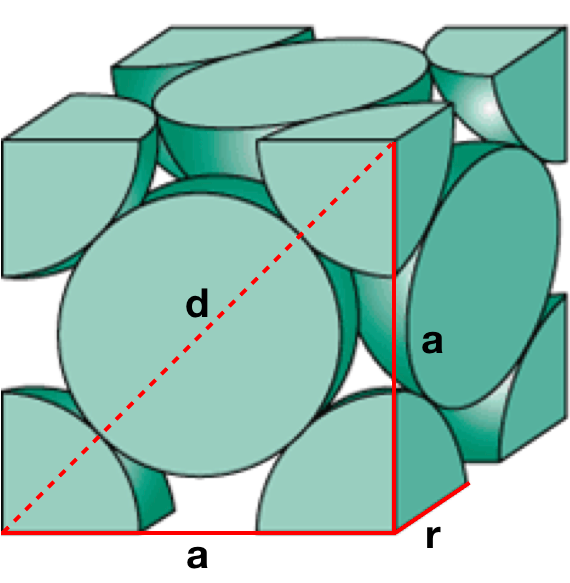# Problem: Aluminum (atomic radius = 1.43 Å) crystallizes in a cubic closely packed structure. Calculate the edge length of the face-centered cubic unit cell and the density of aluminum.

###### FREE Expert Solution

We are being asked to calculate the edge length and density of the face-centered cubic unit cell of aluminum (atomic radius = 1.43 Å)

Recall that the face-centered cubic lattice contains an atom in each of the face of the cube and an atom in each of the corners. It looks like this:We are going to do the following steps:

Step 1: Calculate the edge length of the aluminum unit cell

Step 2: Determine the volume of Al atoms in 1 unit cell

Step 3: Determine the mass of Al atoms in 1 unit cell

Step 4: Calculate the density of the aluminum unit cell

99% (128 ratings)###### Problem Details

Aluminum (atomic radius = 1.43 Å) crystallizes in a cubic closely packed structure. Calculate the edge length of the face-centered cubic unit cell and the density of aluminum.

Frequently Asked Questions

What scientific concept do you need to know in order to solve this problem?

Our tutors have indicated that to solve this problem you will need to apply the Unit Cell concept. You can view video lessons to learn Unit Cell. Or if you need more Unit Cell practice, you can also practice Unit Cell practice problems.

What professor is this problem relevant for?

Based on our data, we think this problem is relevant for Professor Gulde's class at UB.

What textbook is this problem found in?

Our data indicates that this problem or a close variation was asked in Chemistry - OpenStax 2015th Edition. You can also practice Chemistry - OpenStax 2015th Edition practice problems.